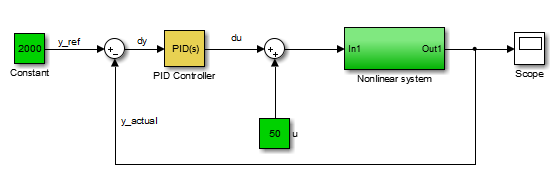## PID 控制的系统辨识

### 线性逼近用 PID 控制的非线性系统

`$\begin{array}{l}\Delta \stackrel{˙}{x}=\frac{\partial f}{\partial x}\Delta x+\frac{\partial f}{\partial u}\Delta u,\\ \Delta y=\frac{\partial g}{\partial x}\Delta x+\frac{\partial g}{\partial u}\Delta u.\end{array}$`### 高级系统辨识任务

PID 调节器中，您只能辨识单输入、单输出、连续时间被控对象模型。此外，PID 调节器无法执行以下系统辨识任务：

• 辨识具有任意数量的极点和零点的传递函数。（PID 调节器可以辨识具有最多三个极点和一个零点以及一个积分器和一个时滞的传递函数。PID 调节器可以辨识任意阶状态空间模型。）

• 估计模型的扰动分量，这对于从噪声动态特性中分离测量的动态特性很有用。

• 通过将被控对象响应与独立数据集进行比较来验证估计。

• 执行残差分析。

## 另请参阅

(System Identification Toolbox)